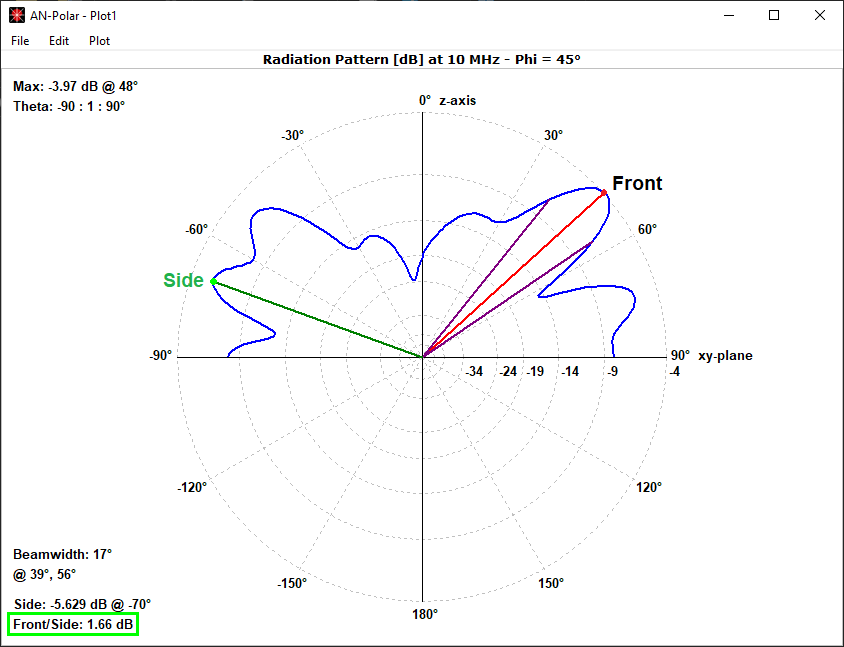How can we help?

# Front-to-Rear and Front-to-Back Ratios

Two commonly used metrics for quantifying the directional properties of an antenna radiation pattern are the front-to-rear ratio, F/R, and the front-to-back ratio, F/B.

The F/R is the ratio of the maximum power radiated by the antenna in the forward direction (toward the front) to the maximum power radiated in the backward direction (toward the rear). This ratio indicates how much stronger the antenna’s radiation is in the forward direction compared to the backward direction. A high F/R indicates that the antenna has a strong directional gain in the forward direction and low radiation in the backward direction.

On the other hand, the F/B is the ratio of the maximum power radiated by the antenna in the forward direction to the power radiated in the opposite direction from the forward direction. In other words, the F/B measures the power ratio between the front of the antenna and the direction directly behind the antenna. A high F/B indicates that the antenna has a strong directional gain in the forward direction and low radiation in the opposite direction.

Figure 1 shows the difference between the F/R (aka worst-case front-to-back ratio) and F/B (aka 180°-front-to-back ratio). Note that these definitions take into account that the radiation pattern slice covers 360 degrees.

In summary, the main difference between the F/R and F/B is the direction of the backward radiation. The F/R measures the power ratio between the front and back of the antenna, while the F/B measures the power ratio between the front and the opposite direction of the antenna.

These definitions are applicable to both horizontal (Theta = const.) and vertical (Phi = const.) radiation patterns in the absence of a ground plane. However, when a ground plane is present, there are some differences to be noted. For horizontal patterns, F/R and F/B are defined in the same way since the angular range covers 360 degrees. On the other hand, when it comes to vertical patterns, the angular range is limited to 180 degrees. In such cases, F/R refers to the ratio of the maximum signal in the pattern to the maximum signal in the opposite quadrant (as shown in Fig. 2) and it is called front-to-side ratio. However, F/B is irrelevant as there is no ‘back’ direction for an infinite horizontal plane, and as a result, AN-SOF will display a value of zero.Fig. 2: Definition of Front-to-Side ratio for a vertical pattern when there is a ground plane.

The Results tab in the AN-SOF main window displays a table depicting F/R and F/B as a function of frequency during a frequency sweep. These values are obtained by analyzing the Vertical (V) and Horizontal (H) slices of the radiation pattern that correspond to the maximum value within the analyzed angular range.

Moreover, in the Plots tab, a chart showcasing F/R and F/B on the same axis is available to facilitate comparison. This chart displays the frequency sweep data in a graphical format for a better understanding of the relationship between F/R and F/B.

Note

• To ensure proper calculations of F/R and F/B, select the Full 3D, Vertical or Horizontal options in the Far-Field panel.
• Selecting the Custom option in the Far-Field panel will lead to variations in the calculation of F/R and F/B as they will depend on the specific angular ranges that have been configured.